#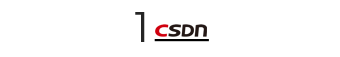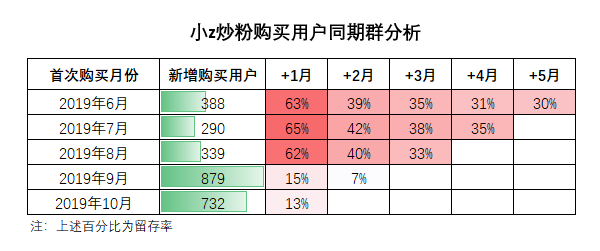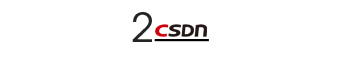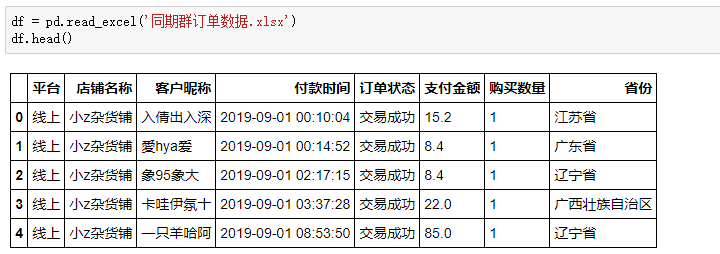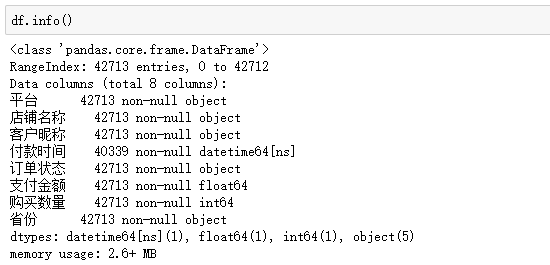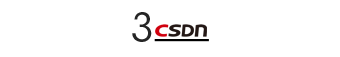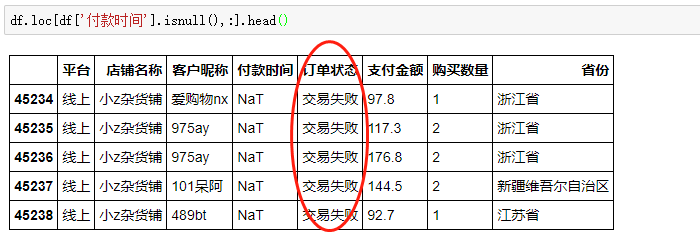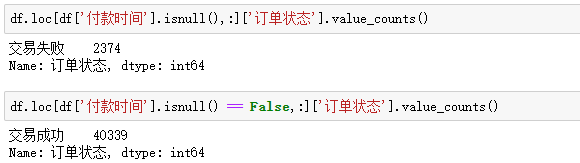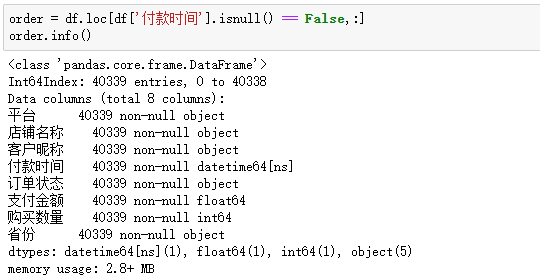40339行数据，就是同期群分析的主战场。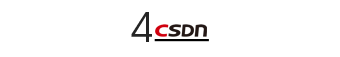• 首先计算出当月新增的客户数，并记录客户昵称；

• 然后拿这部分客户，分别去和后面每个月购买的客户做匹配，并统计有多少客户出现复购（留存）。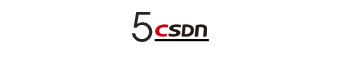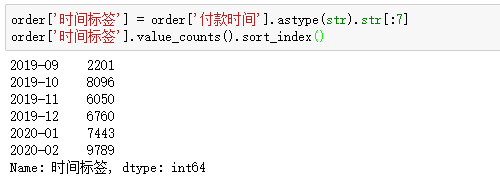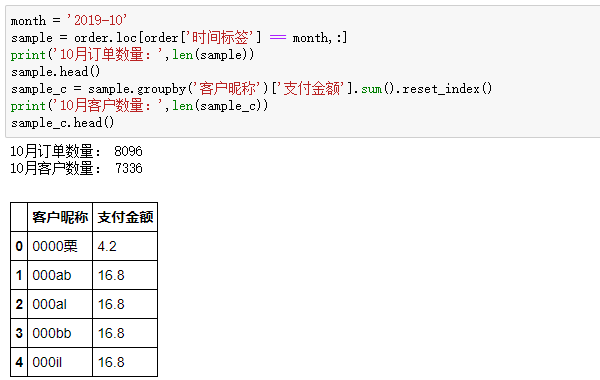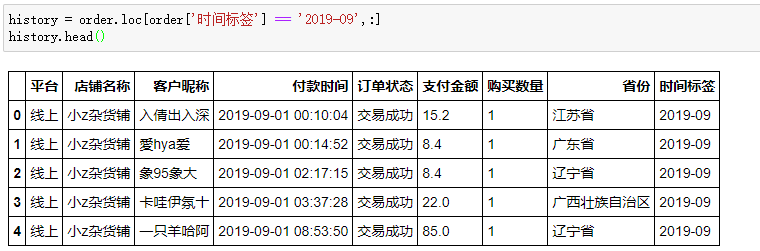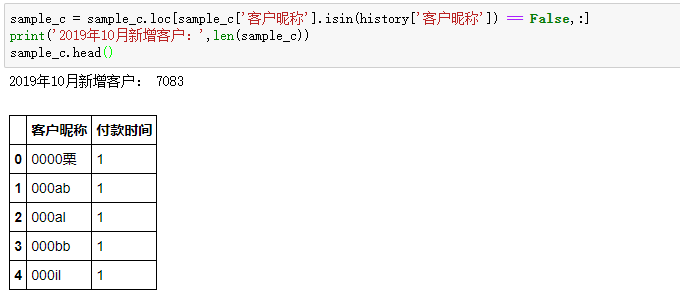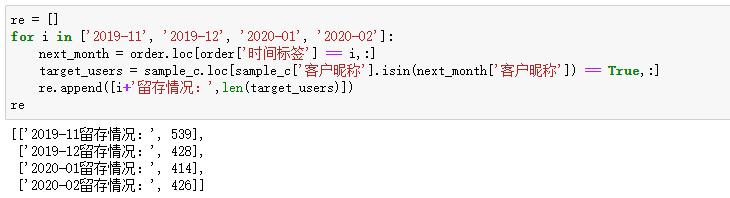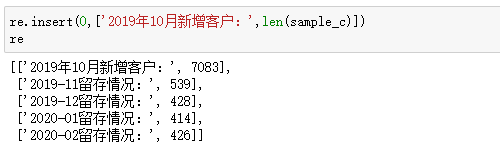2019年10月新增客户7083位，次月（11月）留存539人，随后有所降低，而到了2020年2月留存回购客户数较上月有小幅上升。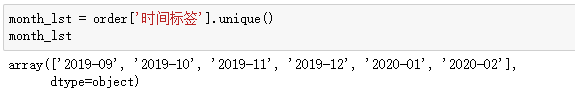``````#引入时间标签
month_lst = order['时间标签'].unique()
final = pd.DataFrame()

for i in range(len(month_lst) - 1):

#构造和月份一样长的列表，方便后续格式统一
count =  * len(month_lst)

#筛选出当月订单，并按客户昵称分组
target_month = order.loc[order['时间标签'] == month_lst[i],:]
target_users = target_month.groupby('客户昵称')['支付金额'].sum().reset_index()

#如果是第一个月份，则跳过（因为不需要和历史数据验证是否为新增客户）
if i == 0:
new_target_users = target_month.groupby('客户昵称')['支付金额'].sum().reset_index()
else:
#如果不是，找到之前的历史订单
history = order.loc[order['时间标签'].isin(month_lst[:i]),:]
#筛选出未在历史订单出现过的新增客户
new_target_users = target_users.loc[target_users['客户昵称'].isin(history['客户昵称']) == False,:]

#当月新增客户数放在第一个值中
count = len(new_target_users)

#以月为单位，循环遍历，计算留存情况
for j,ct in zip(range(i + 1,len(month_lst)),range(1,len(month_lst))):
#下一个月的订单
next_month = order.loc[order['时间标签'] == month_lst[j],:]
next_users = next_month.groupby('客户昵称')['支付金额'].sum().reset_index()
#计算在该月仍然留存的客户数量
isin = new_target_users['客户昵称'].isin(next_users['客户昵称']).sum()
count[ct] = isin

#格式转置
result = pd.DataFrame({month_lst[i]:count}).T

#合并
final = pd.concat([final,result])

final.columns = ['当月新增','+1月','+2月','+3月','+4月','+5月']``````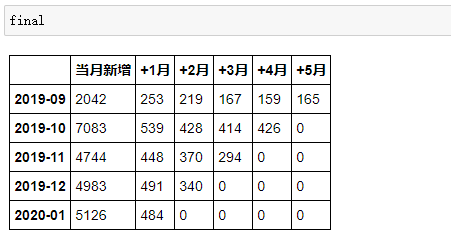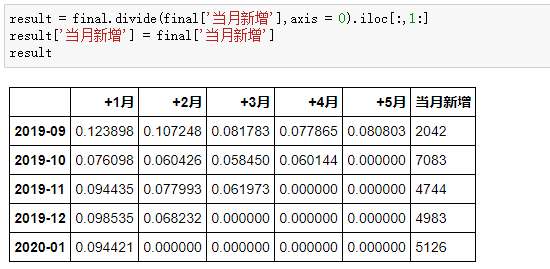• 横向观察，次月流失严重，表现最好的月份次月留存也只有12%，随后平稳降低，稳定在6%左右。

• 纵向对比，2019年当月新增客户最少，仅有2042位，但人群相对精准，留存率表现优于其他月份。

• ...

【End】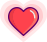点击阅读原文，参与报名